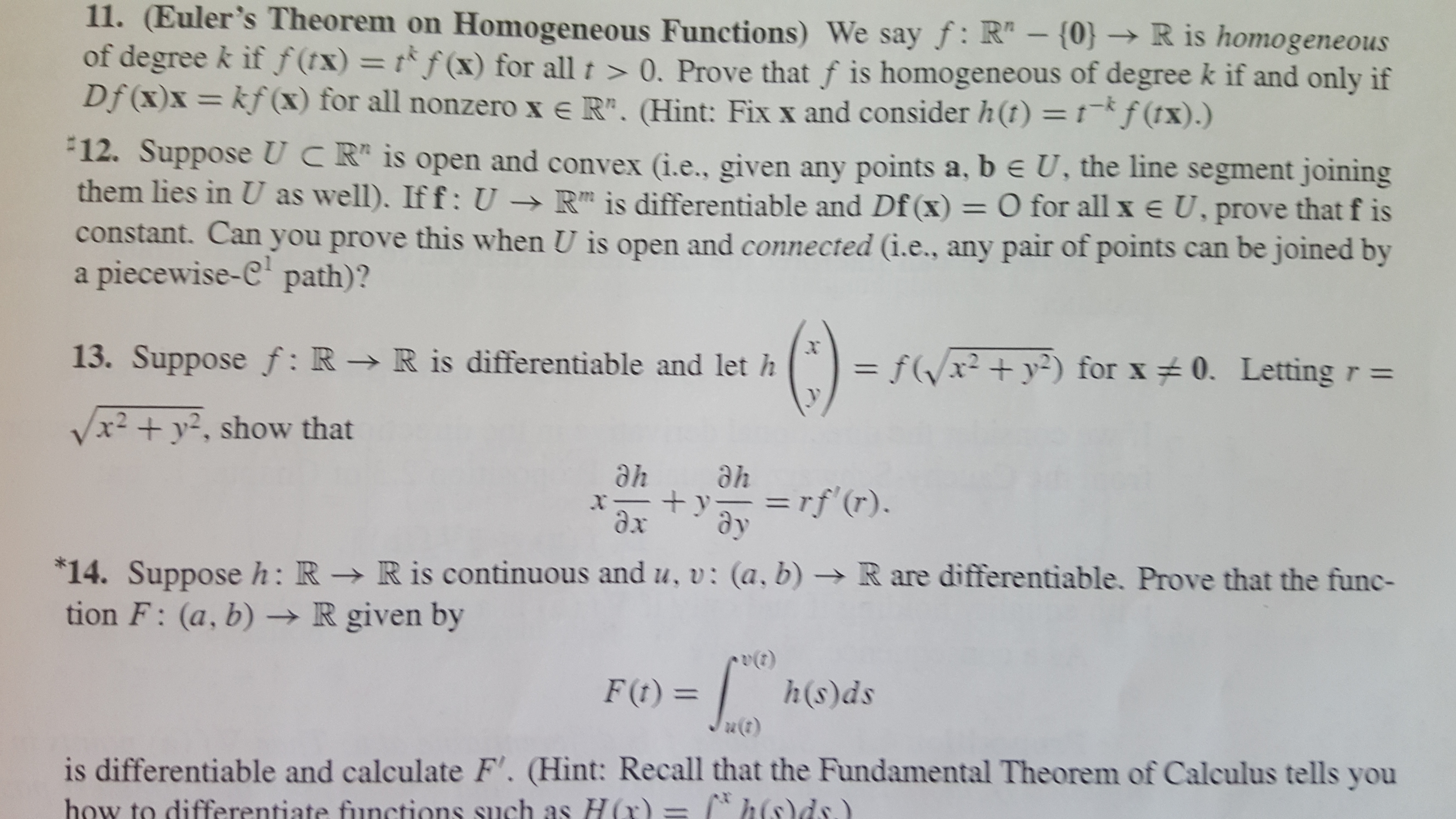# 11. (Euler's Theorem on Homogeneous Functions) We say f: R"- {0} R is homogeneous of degree k if f(tx) = tf(x) for all t >0. Prove that f is homogeneous of degree k if and only if Df (x)x= kf(x) for all nonzero x e R". (Hint: Fix x and consider h(t) = 1-*f (tx) .) 12. Suppose UCR is open and convex (i.e., given any points a, b e U, the line segment joining them lies in U as well). If f: U -R is differentiable and Df (x) = O for all x e U, prove that f is constant. Can you prove this when U is open and connected (i.e., any pair of points can be joined by piecewise-C path)? a 13. Suppose f: R > R is differentiable and let h fxy2) for x 0. Letting r = /x2 +y2, show that ahe he =rf(r). x dx 14. Suppose h: R - R is continuous and u, v: (a, b) tion F: (a, b) -R given by R are differentiable. Prove that the func- h(s)ds F(t)= is differentiable and calculate F'. (Hint: Recall that the Fundamental Theorem of Calculus tells you how to differentiate functions such as H (r) = 2

Question

Hello,

Please help me solve number 13 in the attachment.

Thank you very much.help_outlineImage Transcriptionclose11. (Euler's Theorem on Homogeneous Functions) We say f: R"- {0} R is homogeneous of degree k if f(tx) = tf(x) for all t >0. Prove that f is homogeneous of degree k if and only if Df (x)x= kf(x) for all nonzero x e R". (Hint: Fix x and consider h(t) = 1-*f (tx) .) 12. Suppose UCR is open and convex (i.e., given any points a, b e U, the line segment joining them lies in U as well). If f: U -R is differentiable and Df (x) = O for all x e U, prove that f is constant. Can you prove this when U is open and connected (i.e., any pair of points can be joined by piecewise-C path)? a 13. Suppose f: R > R is differentiable and let h fxy2) for x 0. Letting r = /x2 +y2, show that ahe he =rf(r). x dx 14. Suppose h: R - R is continuous and u, v: (a, b) tion F: (a, b) -R given by R are differentiable. Prove that the func- h(s)ds F(t)= is differentiable and calculate F'. (Hint: Recall that the Fundamental Theorem of Calculus tells you how to differentiate functions such as H (r) = 2 fullscreen

## Expert Answer

1 Rating

### Want to see this answer and more?

Experts are waiting 24/7 to provide step-by-step solutions in as fast as 30 minutes!*

*Response times may vary by subject and question complexity. Median response time is 34 minutes for paid subscribers and may be longer for promotional offers.
Tagged in
Math
Advanced Math© 2021 bartleby. All Rights Reserved.